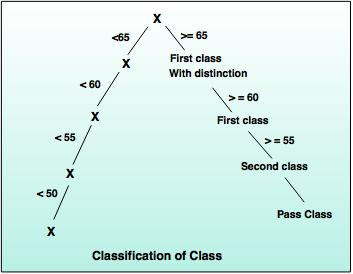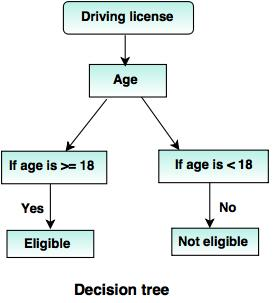# Classification in Data Mining

## Introduction

• Classification constructs the classification model by using training data set.
• Classification predicts the value of classifying attribute or class label.
For example: Classification of credit approval on the basis of customer data.
University gives class to the students based on marks.
• If x >= 65, then First class with distinction.
• If 60<= x<= 65, then First class.
• If 55<= x<=60, then Second class.
• If 50<= x<= 55, then Pass class.## Classification Requirements

The two important steps of classification are:

1. Model construction
• A predefine class label is assigned to every sample tuple or object. These tuples or subset data are known as training data set.
• The constructed model, which is based on training set is represented as classification rules, decision trees or mathematical formulae.
2. Model usage
• The constructed model is used to perform classification of unknown objects.
• A class label of test sample is compared with the resultant class label.
• Accuracy of model is compared by calculating the percentage of test set samples, that are correctly classified by the constructed model.
• Test sample data and training data sample are always different.

## Classification vs Prediction

ClassificationPrediction
It uses the prediction to predict the class labels.It is used to assess the values of an attribute of a given sample.
For example: If the patients are grouped on the basis of their known medical data and treatment outcome, then it is considered as classification.For example: If a classification model is used to predict  the treatment outcome for a new patient, then it is prediction.

## Issues related to Classification and Prediction

1. Data preparation
Data preparation consist of data cleaning, relevance analysis and
data transformation.

2. Evaluation of classification methods
i)  Predictive accuracy: This is an ability of a model to predict the class label of a new
or previously unseen data.
ii) Speed and scalability: It refers to the time required to construct and use the model and increase efficiency in disk- resident databases.

3. Inter-predictability:
It is an understanding and insight provided by the model.

## Decision Tree Induction Method

Decision tree:
• A decision tree performs the classification in the form of tree structure. It breaks down the dataset into small subsets and a decision tree can be designed simultaneously.
• The final result is a tree with decision node.
For example:
The following decision tree can be designed to declare a result, whether an applicant is eligible or not eligible to get the driving license.## Attribute selection methods

#### 1. Gini Index (IBM intelligent Miner)

• Gini index is used in CART (Classification and Regression Trees), IBM's Intelligent Miner system, SPRINT (Scalable Parallelizable Induction of decision Trees).
If a data set 'T' contains examples from 'n' classes, gini index, gini (T) is defined as:After splitting T into two subsets T1,  T2  with sizes N1 and N2, the gini index of the split data is defined as:
ginisplit (T) = N1/ N2 gini (T1) + N2/ N gini (T2)
• For each attribute, each of the possible binary splits is considered. For each attribute, the attribute providing smallest ginisplit (T) is chosen to split the node for continuous- valued attributes, where each possible split-point must be considered.

#### 2. ID3 (Algorithm for inducing a decision Tree)

• Ross Quinlin developed  ID3 algorithm in 1980.
• C4.5 is an extension of ID3.
• It avoids over-fitting  of the data.
• It determines the depth of decision tree and reduces the error pruning.
• It also handles continuous value attributes. For example: salary or temperature.
• It works for missing value attribute and handles suitable attribute selection measure.
• It gives better efficiency of computation.
Algorithm to generate a decision tree from the training tuples of data partition, D.
Step 1: Create a node 'N':
Step 2: If tuple in D are all of the same class, 'C', then go to step 3
Step 3: Return 'N' as a leaf node labeled with the majority class in 'C'
Step 4: If attribute list is empty, then return 'N' as a leaf node labeled with the majority class in D.
Step 5: Apply attribute_selection_method (D, attribute _list) to find the “best” splitting criteria.
Step 6: If splitting_attribute is discrete-valued and multi way, splits are allowed. Then follow step 7
Step 7: Attribute_list ← attribute_list – splitting_attribute;// remove splitting attribute.
Step 8: For each outcome j of splitting creation, Let Dj be the set of data tuples in D that satisfies outcome j, If Dj is empty, then attached leaf is labeled with the majority class in D to node N;
Step 9: Else, attach the node returned by Generate_decision_tree (Dj, attribute_list ) to node N;
Step 10: Return N;
Step 11: Stop.

#### 3. Tree Pruning

• To avoid the overfitting problem, it is necessary to prune the tree.
• Generally, there are two possibilities while constructing a decision tree. Some record may contain noisy data, which increases the size of the decision tree. Another possibility is, if the number of training examples are too small to produce a representative sample of the true target function.
• Pruning can be possible in a top down or bottom up fashion.
Some well known methods to perform pruning are:

1. Reduced error pruning
This is simplest method of pruning. Start from the leaves. Each node is replaced with its most popular class to maintain accuracy.

2. Cost complexity pruning
• It generates a series of trees.
• Consider 'T0' as the initial tree and 'Tm' as root.
• Consider that the tree is created by removing a subtree from tree i- 1 and replacing it with a leaf node with value chosen as per the tree constructing algorithm.
• The subtree which is removed can be chosen as follows:
• Define the error rate of tree 'T' over data set 'S' as err (T,S).
• The subtree from tree that minimizes is chosen for removal.
• The function (T,t) defines the tree, which is obtained by pruning the subtrees 't' from the tree 'T'. After creating series of tree, the best tree is chosen by measuring a training set or cross-validation.
3.  Alpha-beta pruning
• It is a search algorithm, which improves the minimax algorithm by eliminating branches which will not be able to give further outcome.
• Let alpha (α) be the value of the best choice along the path for higher value as MAX.
• Let beta (β) be the value of the best choice along the path for lower value as MIN.
• While working with decision tree, the problem of missing values (those values which are missing or wrong)  may occur.
• So, one of the most common solution is to label that missing value as blank.

## Prediction

• Prediction deals with some variables or fields, which are available in the data set to predict unknown values regarding other variables of interest.
• Numeric prediction is the type of predicting continuous or ordered values for given input.
For example: The company may wish to predict the potential sales of a new product given with its price.
• The most widely used approach for numeric prediction is regression.